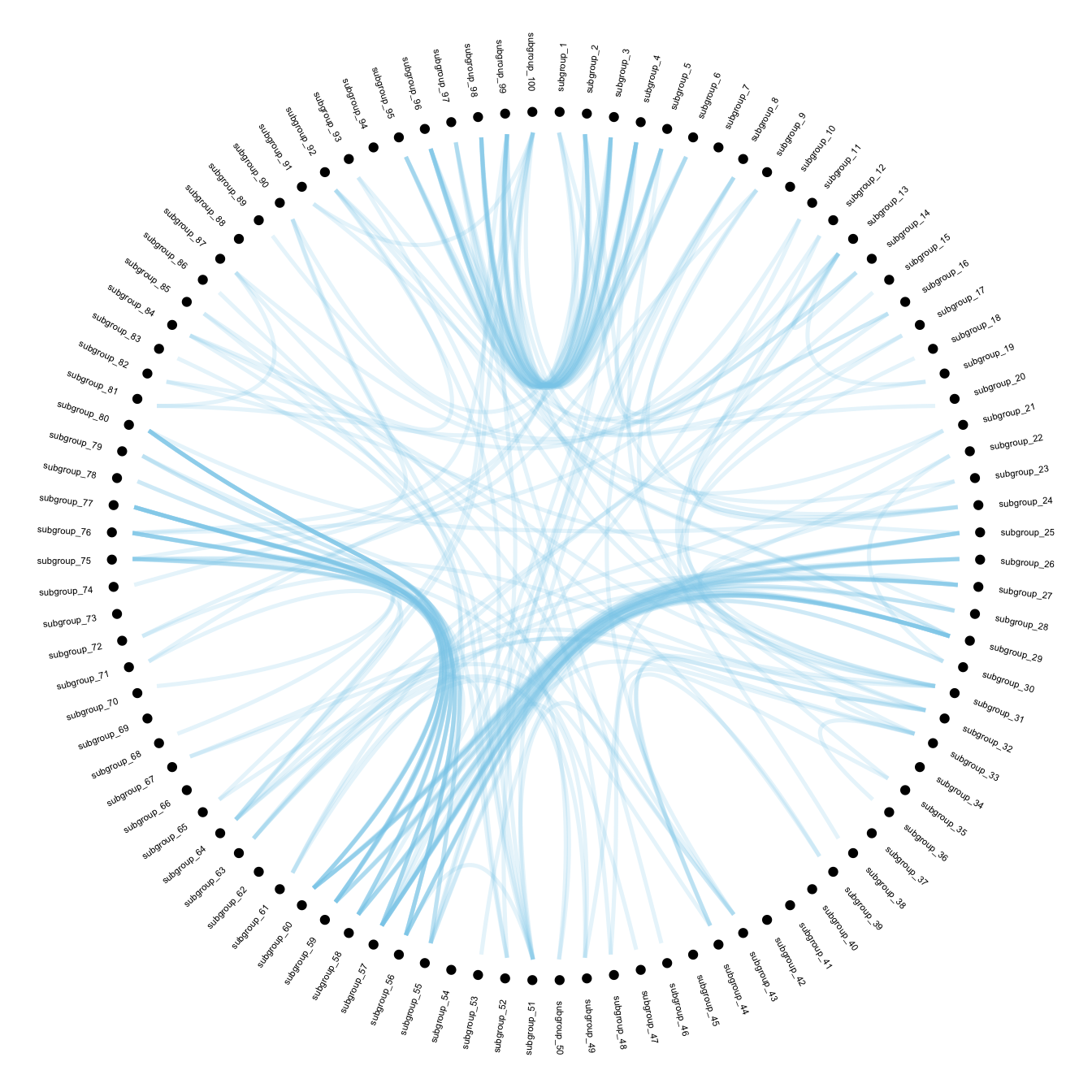# Add labels to Hierarchical Edge Bundling

This post describes how to add labels on a hierarchical edge bundling chart. It explains how to proceed, with reproducible `R` code, using the `ggraph` package.

# Without Labels

Previous post of the hierarchical edge bundling section explained:

Let’s remind how to prepare the data for the `ggraph` library.

``````# Libraries
library(ggraph)
library(igraph)
library(tidyverse)
library(RColorBrewer)

# create a data frame giving the hierarchical structure of your individuals
set.seed(1234)
d1 <- data.frame(from="origin", to=paste("group", seq(1,10), sep=""))
d2 <- data.frame(from=rep(d1\$to, each=10), to=paste("subgroup", seq(1,100), sep="_"))
edges <- rbind(d1, d2)

# create a dataframe with connection between leaves (individuals)
all_leaves <- paste("subgroup", seq(1,100), sep="_")
connect <- rbind(
data.frame( from=sample(all_leaves, 100, replace=T) , to=sample(all_leaves, 100, replace=T)),
data.frame( from=sample(head(all_leaves), 30, replace=T) , to=sample( tail(all_leaves), 30, replace=T)),
data.frame( from=sample(all_leaves[25:30], 30, replace=T) , to=sample( all_leaves[55:60], 30, replace=T)),
data.frame( from=sample(all_leaves[75:80], 30, replace=T) , to=sample( all_leaves[55:60], 30, replace=T)) )
connect\$value <- runif(nrow(connect))

# create a vertices data.frame. One line per object of our hierarchy
vertices  <-  data.frame(
name = unique(c(as.character(edges\$from), as.character(edges\$to))) ,
value = runif(111)
)
# Let's add a column with the group of each name. It will be useful later to color points
vertices\$group  <-  edges\$from[ match( vertices\$name, edges\$to ) ]``````

# Create the labels

Next step: computing the label features that will be displayed all around the circle, next to the nodes:

• angle → vertical on top and botton, horizontal on the side, and so on.
• flip it → labels on the left hand side must be 180° flipped to be readable
• alignment → if labels are flipped, they must be right aligned

Those information are computed and added to the `vertices` data frame.

``````#Let's add information concerning the label we are going to add: angle, horizontal adjustement and potential flip
#calculate the ANGLE of the labels
vertices\$id <- NA
myleaves <- which(is.na( match(vertices\$name, edges\$from) ))
nleaves <- length(myleaves)
vertices\$id[ myleaves ] <- seq(1:nleaves)
vertices\$angle <- 90 - 360 * vertices\$id / nleaves

# calculate the alignment of labels: right or left
# If I am on the left part of the plot, my labels have currently an angle < -90
vertices\$hjust <- ifelse( vertices\$angle < -90, 1, 0)

# flip angle BY to make them readable
vertices\$angle <- ifelse(vertices\$angle < -90, vertices\$angle+180, vertices\$angle)``````

# Plot the labels

Now that label features have been computed, we just need to display it on the chart using the `geom_node_text()` function.``````# Create a graph object
mygraph <- igraph::graph_from_data_frame( edges, vertices=vertices )

# The connection object must refer to the ids of the leaves:
from  <-  match( connect\$from, vertices\$name)
to  <-  match( connect\$to, vertices\$name)

# Basic usual argument
ggraph(mygraph, layout = 'dendrogram', circular = TRUE) +
geom_node_point(aes(filter = leaf, x = x*1.05, y=y*1.05)) +
geom_conn_bundle(data = get_con(from = from, to = to), alpha=0.2, colour="skyblue", width=0.9) +
geom_node_text(aes(x = x*1.1, y=y*1.1, filter = leaf, label=name, angle = angle, hjust=hjust), size=1.5, alpha=1) +
theme_void() +
theme(
legend.position="none",
plot.margin=unit(c(0,0,0,0),"cm"),
) +
expand_limits(x = c(-1.2, 1.2), y = c(-1.2, 1.2))``````

# With Customization

To get the final figure, it is necessary to add customization described in graph #310:

• control node size, color and transparency.
• control connection color
``````ggraph(mygraph, layout = 'dendrogram', circular = TRUE) +
geom_conn_bundle(data = get_con(from = from, to = to), alpha=0.2, width=0.9, aes(colour=..index..)) +
scale_edge_colour_distiller(palette = "RdPu") +

geom_node_text(aes(x = x*1.15, y=y*1.15, filter = leaf, label=name, angle = angle, hjust=hjust, colour=group), size=2, alpha=1) +

geom_node_point(aes(filter = leaf, x = x*1.07, y=y*1.07, colour=group, size=value, alpha=0.2)) +
scale_colour_manual(values= rep( brewer.pal(9,"Paired") , 30)) +
scale_size_continuous( range = c(0.1,10) ) +

theme_void() +
theme(
legend.position="none",
plot.margin=unit(c(0,0,0,0),"cm"),
) +
expand_limits(x = c(-1.3, 1.3), y = c(-1.3, 1.3))``````Related chart types

## Contact

This document is a work by Yan Holtz. Any feedback is highly encouraged. You can fill an issue on Github, drop me a message on Twitter, or send an email pasting yan.holtz.data with gmail.com.